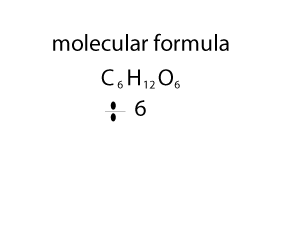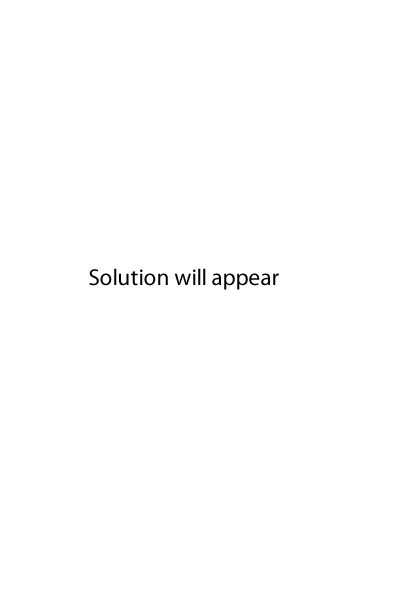Empirical to molecular formulae By experimentation we can work out the empirical formula of many compounds. It is only a small step away to obtaining the molecular formula. As you can see form the animation on the right, the empirical and molecualr formula differ by a certain number. In the case of glucose (C6H12O6 ) that number is 6. If we divide the molecular formula by 6 we get the empirical formula and if we mulitply the empirical formula by 6 we get the molecular formula.So how do we change an empirical formula to a molecular formula? We multiply the empirical formula by a number derived by dividing the molecular mass by the empirical mass, as shown on the right.A hydrocarbon is composed of 85.7% carbon. If 1.2 X 1023 molecules of this hydrocarbon weigh 33.6 grams find the molecular formula. First find the empirical formula of the compound. - Step 1 convert the mass of each element into mol. - Step 2 find the simplest ratio and deduce the empirical formula Second find the number the empirical formula must be multiplied by to get the molecular formula. Find the molecular formula Try the exercises below. A compound with a molar mass of 34.0 g/mol contains 5.88% hydrogen and 94.12% oxygen by mass. Find the molecular formula for this compound. Solution Naphthalene has a molar mass of 128g/mol. It contains 93.75% C and 6.25% hydrogen. Find its molecular formula. An organic acid present in oranges has a percentage composition, by mass, of 37.5% C, 58.3% O and 4.2% H. If its molar mass is 192 g/mol find the molecular formula. A compound has a molecular mass of 62. Its percentage composition, by mass, is found to be 38.7% C, 9.7% H and 51.6% C. Find its molecular formula.